## 5 Insights From Taking A Live Cohort-Based Course (And How I’m Applying Them To My Own)

I recently joined 40 other entrepreneurs for the first cohort of Billy Broas’ new live online cohort-based course, the Keystone Accelerator.

It’s an 8-week program covering how to market and launch cohort-based courses (CBCs).

Cohort-based courses are online courses where a group of students join at the same time and progress through the course together. Typically, students in a CBC meet online via Zoom for interactive sessions and work on course materials between sessions.

They’re fundamentally different to self-paced video courses because the emphasis shifts from being content-centric to being community-centric.

Students get the benefit of accountability, a peer group and expert teacher(s) for questions, and a community in which to celebrate wins and share challenges. CBC’s provide structure and guidance for students. Consequently, they have much higher completion rates than self-paced courses and better outcomes for students.

I joined this Keystone cohort course because I’m creating my own live training course and I wanted to learn from the experts on how to build, market and sell these types of courses.

We met twice a week for 90 minutes over Zoom – with participants calling in from all over the world – to learn an education-based marketing framework. In addition, I joined weekly peer coaching groups to brainstorm ideas and critique each other’s work in a safe environment.

Here are my top five takeaways from taking a cohort-based course that I want apply to my own course, the Pro Sheets Accelerator:

## 1. It Is Possible To Have Transformative Experiences Online

I’d never done a live cohort-based course before Keystone. I’d experienced plenty of webinars, in-person courses and self-paced video courses.

But this was different.

Keystone was unlike any other learning experience I’ve had.

It combined the content of an on-demand video course with the accountability, rapid feedback and community of an in-person event.

Accountability pushes you to show up and do the work.

Rapid feedback means you don’t get stuck for long periods and learn quickly from your mistakes.

Community provides a safe space to share wins and challenges and make new friends.

Combining these three factors with world-class content is the best way to facilitate the student transformation.

For first cohort of the Pro Sheets Accelerator, I want to foster a really strong community to maximize these benefits.

If you join, you’ll be in a group of 30 – 40 students, with a private course forum, peer groups and office hours, in addition to the main teaching sessions.

## 2. The Connections You Make Are As Valuable As The Course Content

I joined a group of 40 super smart, motivated entrepreneurs, all building their own live training courses in wildly different industries.

Some were earlier in their journeys than me, some were further along.

Together, the breadth of experiences, ideas and insights far surpassed what I, as an individual, could have achieved.

Truly a case of the whole being greater than the sum of its parts.

I learned a lot from the peer coaching sessions, both from giving and receiving feedback. Seeing how other people solve problems, how they think and how they approach opportunities and challenges, was hugely valuable.

Like a wifi network that gets stronger as more nodes are added and interlinked, the new connections you make through a CBC improve your chances of success with your own business.

## 3. Learning With Others Is More Fun Than Learning Alone

I’m a firm believer that education should be enjoyable and that you learn best when you’re having fun. We should take inspiration from young children who learn through play.

If it’s enjoyable, you’re more likely to succeed.

Instead of watching the clock and counting down until the end of a boring lecture, you’re emotionally present and absorbing information. You’re in the zone of proximal development.

In the past year, we’ve all been starved of human connection and friendship.

Although CBCs are online, you’re still going through an intense shared experience with other folks.

Naturally friendships form as people get to know each other and become more comfortable. You begin to see beneath the surface and really learn from each other.

The Pro Sheets Accelerator will be a fun experience. My goal is for everyone to enjoy the course first and foremost.

## 4. The Tangential Ideas Are Just As Valuable As The Core Content

The Keystone course I participated in is a marketing course. Its promise is that it’ll teach you how to market and sell cohort-based courses.

But along the way, I picked up tons of other ideas. Ideas that weren’t on the syllabus but arose through digressions, chats with peers or from surprise bonus sessions with guest experts.

Simply from being part of this cohort-based course, I learned about how to run one. I saw first hand how many moving pieces there are and why you need help to run one. Everything from the first onboarding call through to how to structure the live Zoom calls and exercises.

Tiago Forte, one of the world’s leading experts on CBCs who runs the hugely successful Building A Second Brain course, joined this Keystone course and added his perspective to the program.

In addition, we had workshops on the operations side of running CBCs from course director Will Mannon, and on how to create engaging experiences for our students from learning designer Andrew Barry. Both of these added valuable insights outside of the marketing curriculum of the main course.

Encouraging curiosity, fostering peer-led learning and surprising students with guest speakers are all great ways to add value to a CBC.

I plan to implement all of these in the Pro Sheets Accelerator course.

## 5. Less Is More in Cohort-Based Courses

When it comes to content, less is more.

What this means in practice is that the emphasis of the course shifts from cramming in as much content as possible (a traditional signal of value) to focusing on students’ transformational learning experiences.

The goal of a CBC is for your students’ life to change. You’re guiding them across the bridge from their current status quo to the new, better life.

For example, in the Keystone course, I came away with a much deeper understanding of CBCs and education based marketing. I now have a playbook I can apply to my own business.

What I don’t have is a library of 300 videos on marketing, which I would never have the time to watch, much less implement.

This realization with regards to content – that less is more – was a key shift I noticed in myself during this Keystone course.

For the Pro Sheets Accelerator cohort-based course, my original plan was to focus on making it as comprehensive as possible, covering Google Sheets and Apps Script from top to bottom.

It would have been impossible to achieve and unmanageable for students.

Now, my plan is to focus on two areas:

1. Developing a framework and skillset for doing data analysis with Google Sheets, and
2. Automating that framework

Yes, they’re still big topics, but they’re focussed. I can build a syllabus that goes deep into these subjects and delivers huge value, in a way that won’t overwhelm students.

The material will be relevant. Students will learn just enough to experience a transformation but not too much that it gets diluted.

## Applying These Lessons To Pro Sheets Accelerator

It’s been 4 years since I launched my first online course, How To Build Dashboards in Google Sheets.

Since then, over 40,000 students from 1,000s of organizations have registered for one or more of my online courses.

All of these courses are self-serve video courses.

Now it’s time to add a new experience-based course into the mix, as the next evolution of my education business.

I’m building a cohort-based course called Pro Sheets Accelerator, which will teach you how to leverage the power of data and automation in Google Sheets to grow your business and career.

The first cohort begins at the end of April. I’m super excited to bring together a group of Sheets aficionados for a transformative learning experience. Join us!

## PI Function in Google Sheets And Other Fun π Facts

The PI function in Google Sheets returns the value of the mathematical constant pi (π) to 9 decimal places: 3.141592654

Pi is defined as the ratio of a circle’s circumference to its diameter.

Pi is denoted by the Greek lowercase letter π

## PI Function in Google Sheets: Syntax

`=PI()`

There are no arguments. If you put anything between the brackets the formula will give you an error.

## Circle Calculations With The PI Function

If you know the circumference of a circle then you can calculate the diameter and vice versa.

### How to calculate the circumference

Suppose you have a diameter of 10 (the units are irrelevant).

Then you calculate the circumference with this formula: the diameter multiplied by pi.

`= 10 * PI()`

### How to calculate the diameter

Now suppose you know the circumference is value 10.

The diameter is calculated as the circumference divided by pi:

`= 10 / PI()`

### How to calculate the area of a circle

The formula for the area of a circle is π * r² (pi times the radius squared).

To calculate the radius, you must divide the diameter by 2.

So, knowing the diameter d, you can calculate the area of a circle as follows: π * (d/2)²

Taking a diameter of 10, the area is:

`= PI() * ( 10 / 2 )^2`

## Creating the PI Symbol with the CHAR Function

The amazing CHAR function, which converts numbers into characters per the Unicode table, can output the symbol for pi in your Google Sheet.

Use this formula to output the pi symbol π:

`=CHAR(960)`

Setting the cell to the Merriweather font gives it the nice rounded appearance shown in this image: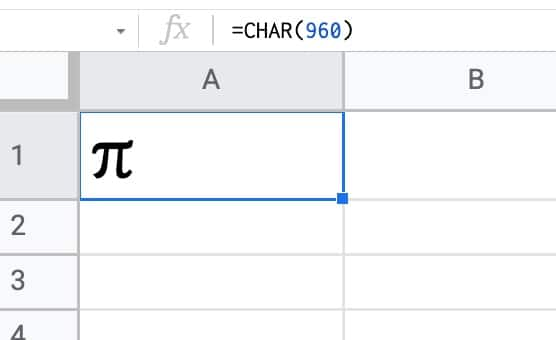By the way, this formula:

`=CHAR(129383)`

will output an image of an entirely different type of pie in your Google Sheet: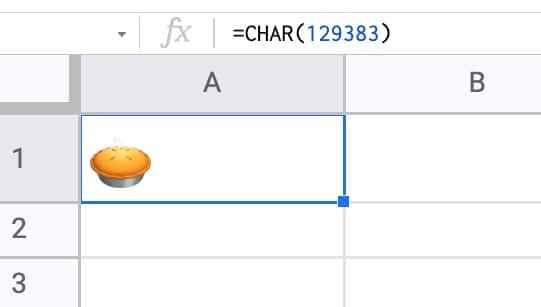## PI function template

Feel free to make a copy: File > Make a copy…

If you can’t access the template, it might be because of your organization’s Google Workspace settings. If you click the link and open in an Incognito window you’ll be able to see it.

## Approximating Pi in Google Sheets With the Nilakantha Series

The Nilakantha series, named after the 15th century mathematician of the same name, is an infinite series that converges on pi as the number of iterations rises. It converges quickly so it’s a good algorithm to use to compute the digits of pi.

The Nilakantha series is:

Let’s build an approximation of pi in a Google Sheet using this series.

### Nilakantha Series in Google Sheets With The PI Function

In cell A1, enter this SEQUENCE formula:

`=SEQUENCE(1000,1,2,2)`

And in B1:

`=SEQUENCE(1000,1,3,2)`

Then in C1:

`=SEQUENCE(1000,1,4,2)`

These three formulas give us three columns of numbers with 1,000 digits each. They start with 2, 3 and 4 respectively and increment by 2.

Look closely at the infinite series definition above and you’ll notice that each row represents a denominator for each iteration of the series.

In cell D1, multiply the values together for each row:

`=PRODUCT(A1:C1)`

Copy this formula down the column.

This formula generates the denominators for the series.

In cell E1, put this product as the denominator to calculate the series values:

`= 4 / D1`

Next, we need to alternate adding and subtracting these values, which we can do with this formula:

`=IF( ISODD( ROW() ), E1 , UMINUS( E1 ) )`

This IF formula checks if we’re on an odd numbered row (e.g. row 1) using the ISODD function and, if we are, returns the number unchanged. But if we’re on an even numbered row, it returns the negative value from column E, using the UMINUS function.

Finally we can approximate pi in column G.

In G1, put this formula to start the series:

`= 3 + F1`

In cell G2, put this formula:

`= G1 + F2`

and drag this formula all the way to the bottom of the range.

With 1,000 rows, we get a value for pi of 3.1415926533, which is a similar level of accuracy to the PI function.

Here are the first 16 iterations, the remaining rows up to 1,000 can be found in the template linked above in this article: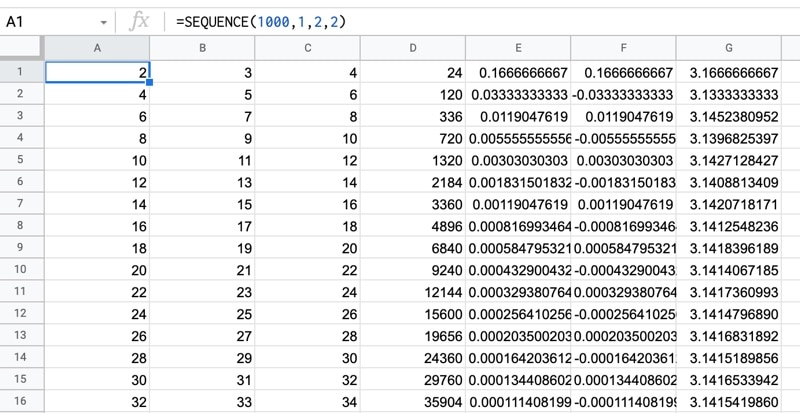Now, you might be wondering how many digits of pi have been calculated…

…well it’s a whopping 31.4 trillion digits!

Obviously, that is not a calculation you can perform in your Google Sheet, but interestingly, it was performed on Google Cloud infrastructure!

Update: The record is now 50 trillion digits, broken by an enthusiast with a self-built server setup. Super impressive!

## Getting More Digits of Pi in Google Sheets

Whilst we can’t get 50 trillion digits of pi into a Google Sheet, we can call the Pi Delivery API and retrieve more digits.

Here’s the code to request the first 1,000 digits of pi and display them in a Google Sheet as a text string (because numbers get truncated):

```/**
* function to call the Pi API
* https://pi.delivery/#apipi_get
*/
function getPI() {

// endpoint
const root = 'https://api.pi.delivery/v1/pi';

// start point and number of digits
const startDigit = 0;
const numDigits = 1000; // max request is 1000 digits in 1 call

// create endpoint
const endpoint = root + '?start=' + startDigit + '&numberOfDigits=' + numDigits;

// call the PI API to fetch digits of pi
const response = UrlFetchApp.fetch(endpoint);
const result = JSON.parse(response.getContentText());

// get pi
// keep pi as a string otherwise it gets truncated if a number
// add single tick in front of the 3 to avoid automatic conversion to number in Sheet
const piDigits = result.content;
const pi = "'3." + piDigits.substring(1);

// paste result into Google Sheet
const piSheet = ss.getSheetByName('PI API');
piSheet.getRange(3,1).setValue(pi);

}
```

The API is limited to calls of up to 1,000 digits at a time, so if you want more than that you’ll need to expand the script above to request batches of 1,000.

## More Resources For Mathematics in Google Sheets

How To Draw The MandelBrot Set In Google Sheets, Using Only Formulas

## INDIRECT Function in Google Sheets

The INDIRECT function in Google Sheets is used to convert text strings into valid cell or range references.

For example, the INDIRECT function will convert the text string “A1” into the cell reference A1. The formula is:

`=INDIRECT("A1")`

which is equivalent to this formula:

`= A1`

It gives the answer 10 in the following example because that’s the value in cell A1: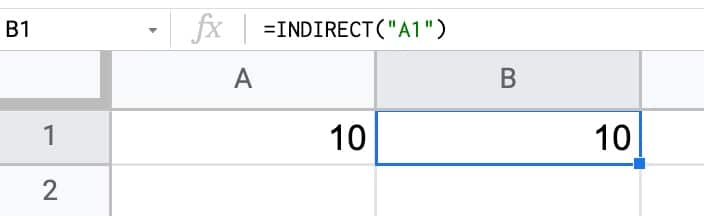## INDIRECT Function in Google Sheets: Syntax

`=INDIRECT(cell_reference_as_string, [is_A1_notation])`

It takes two arguments:

1. `cell_reference_as_string`
2. `[is_A1_notation]`

Here is what they mean:

### cell_reference_as_string

The first argument is a text string that represents a cell or range reference, e.g.:

`"A1"`

`"Sheet1!B4:D100"`

It can also point to a cell containing a range reference, as shown here: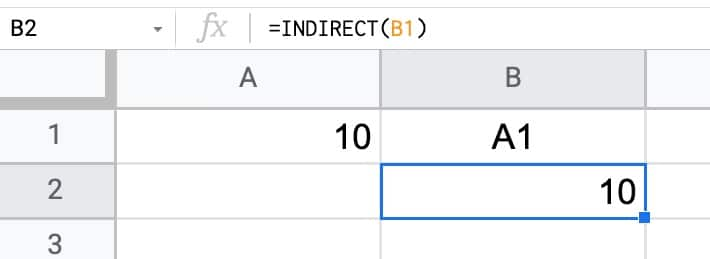The value in cell B1 is the text value “A1”.

When the INDIRECT points to B1, it picks up this string reference and then converts it to an actual cell reference.

### [is_A1_notation]

This second argument is an optional argument that is either TRUE or FALSE.

• If you set it to TRUE, the INDIRECT function to use familiar A1 style notation (e.g. A1, B2:D15, G1:M1000)
• Setting this argument to FALSE forces the INDIRECT function to use the uncommon R1C1 notation (see below)

If you omit this argument, and your INDIRECT just has a single argument like the example at the top of this page, it defaults to A1 notation as if you had used a TRUE argument.

I’ve never seen a formula with this second argument set to FALSE in the wild. So you can almost certainly ignore this argument and just use the INDIRECT function with a single input.

### Aside On R1C1 Notation

R1C1 notation describes a cell or range reference using a row number and column number.

R1C1 is an absolute reference to the cell at position (1,1) in your Sheet, i.e. A1 in regular notation.

The reference with square brackets – RC – is a relative R1C1 reference meaning the cell 1 row down and 1 column to the right of the current cell, wherever that is in your worksheet.

Here’s an example of the INDIRECT using the R1C1 notation: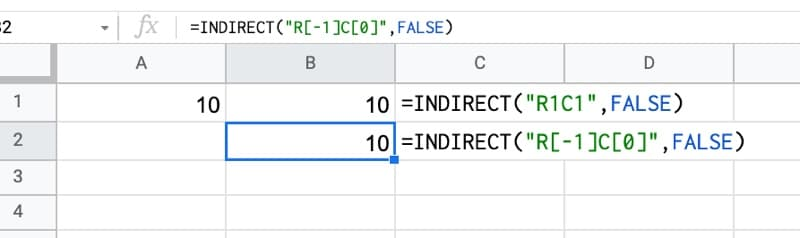Both formulas have FALSE as the last argument of the INDIRECT function, so they’re using R1C1 notation.

The first has the absolute notation – “R1C1” – so it points to cell A1, the cell at Row 1 and Column 1 in your Sheet.

The second – “R[-1]C” – is a relative reference telling the INDIRECT function to go back one row (i.e. the row above, going from row 2 to row 1) and stay in the same column (because the C value is 0).

This is equivalent to the formula:

`= B1`

R1C1 notation can also reference ranges by joining two cell references with a “:” colon, similar to how ranges are referenced in A1 notation, e.g.:

`R[-3]C:R[-1]C`

## INDIRECT function template

Feel free to make a copy: File > Make a copy…

If you can’t access the template, it might be because of your organization’s Google Workspace settings. If you click the link and open in an Incognito window you’ll be able to see it.

The INDIRECT function is also covered in the Day 22 lesson of my free Advanced Formulas 30 Day Challenge course.

## Using The INDIRECT Function To Work With Multiple Sheets

Suppose you have multiple sheets in you Google Sheets file, each with a similar structure. For example, it could be that you have transaction data per year, each in their own sheets: 2018 Data, 2019 Data, 2020 Data, 2021 Data

If you want to perform a calculation across all four sheets at once (e.g. a grand total), you have to click into each sheet from a formula and it’s rather tedious, especially if you have lots of sheets.

By using the INDIRECT function, you can build the formula with strings to save having to click on each tab.

With a list of sheet names in column A, use this INDIRECT formula in column B to access values from each sheet without needing to click across:

`=INDIRECT(A1&"!B6")`

Drag this formula down the column and it will return the value in cell B6 of each sheet: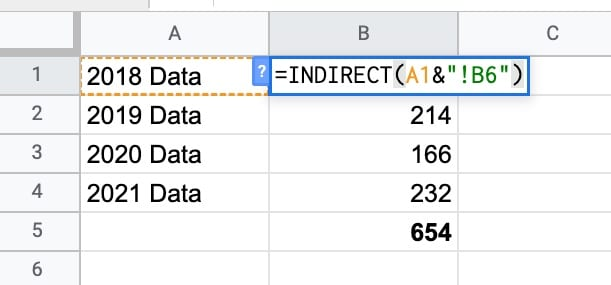The first formula in cell B2 returns the value from cell B6 of the “2018 Data” sheet: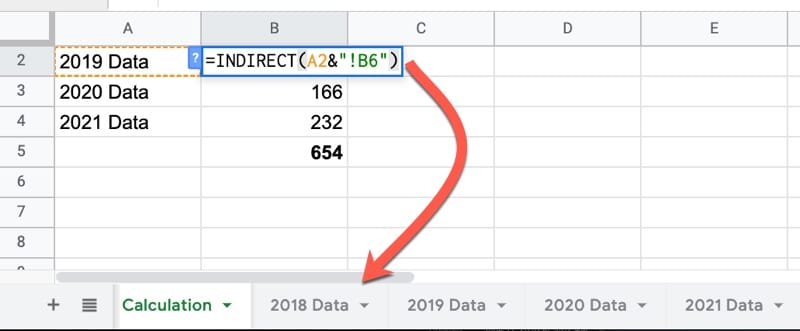## VLOOKUP + INDIRECT Formula in Google Sheets

This is another example of how to use the INDIRECT function to work with multiple sheets.

By combining the VLOOKUP and INDIRECT functions, you can lookup data from different sheets based on a user input.

It requires you to have consistently named tabs in your Google Sheet, e.g. something like Data 2018, Data 2019, Data 2020, Data 2021

Create a data validation drop down to let the user select one of these sheet names and then use the INDIRECT function to convert the text string into a valid range reference which becomes your lookup table.

`=VLOOKUP(A2,INDIRECT(B2&" Data!A1:B5"),2,false)`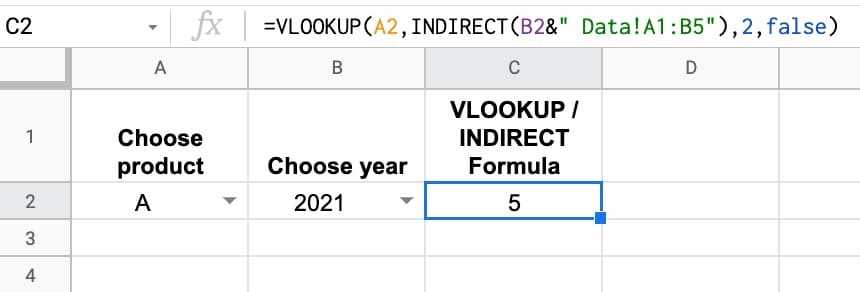## Using The INDIRECT Function To Create Vectors

As you progress in your spreadsheet career, you’ll start to work more frequently with arrays of data rather than single values. One thing you’ll find yourself doing fairly often is needing to create a numbered list.

For example, you might want to sort data on the fly but need some way to get back to the original order. By using a combination of a column vector and array literals {…} you can build this inside the formula without having to add a helper column to your data.

Vectors crop up in other complex formulas too, like this text reverser, unpivot or even advanced sparklines like this clock or this visualize value experiment.

The INDIRECT function can be combined with the ROW function to create column vectors, or combined with the COLUMN function to create row vectors (yes, I realize that sounds strange, but you’ll see what I mean below).

NOTE: These methods have largely been replaced by the simpler, more elegant SEQUENCE function which can easily generate row or column vectors. However, you may still see the INDIRECT version in online forums or older resources, hence why I’m sharing here.

This formula creates a vector from 1 to 10 running down a column:

`=ArrayFormula(ROW(INDIRECT("1:10")))`

This formula creates a vector from 1 to 10 running across a row:

`=ArrayFormula(COLUMN(INDIRECT("A:J")))`The formula can be generalized to create vectors of variable length. One example might be measuring the length of a text string in cell A1 and creating a vector matching that length.

This particular technique as part of a formula to reverse text in Google Sheets (again the newer SEQUENCE function could make this more succinct).

`=ArrayFormula(ROW(INDIRECT("1:"&LEN(A1))))`

The INDIRECT function can also be used to build dynamic named ranges in Google Sheets.

`=SUM(INDIRECT(dynamicRange))`

## IF Function in Google Sheets

The IF function in Google Sheets is used to make decisions with your data. It’s from the Logical family of functions in Google Sheets.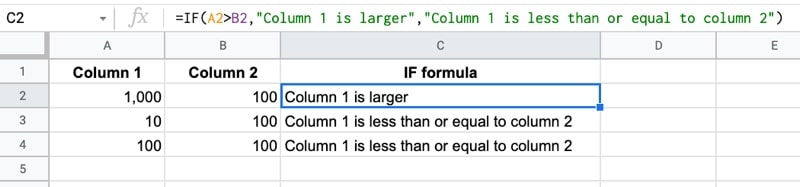In this tutorial, you’ll see how to use IF formulas in Google Sheets to make decisions with your data.

## What does the IF Function do?

At its heart, the IF function is a test that evaluates to a true or false value with a defined behavior if the outcome is true and a different behavior when the outcome is false.

Let’s see an example.

Suppose you have two columns of numbers in A and B and you want to compare each row to see which column has the larger number.

You would use the IF function in Google Sheets to do this!`=IF( A2 > B2 , "Column 1 is larger" , "Column 1 is less than or equal to column 2" )`

Inside the IF formula, the first expression `A2 > B2` checks whether the value in cell A2 is greater than the value in cell B2.

The outcome of this test is either a TRUE or a FALSE value.

The IF function requires a TRUE or FALSE value for this first argument.

Next up, you specify what you want to happen when the result is true. In this example the output of the function in the cell is “Column 1 is larger”

The final argument is the value you want to show if the result is false. In this example, that means the number in column A was smaller (or equal to) the value in column B. In this case, the output of the function is “Column 1 is less than or equal to column 2”.

## IF Function in Google Sheets: Syntax

` =IF(logical_expression, value_if_true, value_if_false)`

You might hear it referred to as an IF function, an IF formula or even an IF statement, but they all mean the same thing.

It takes 3 arguments:

`logical_expression`

An expression that gives a TRUE or FALSE answer, or a cell that contains a TRUE or FALSE value.

`value_if_true`

The value displayed by the IF function if the logical expression has the TRUE value.

`value_if_false`

The value displayed by the IF function if the logical expression has the FALSE value.

## IF function template

Feel free to make a copy: File > Make a copy…

If you can’t access the template, it might be because of your organization’s Google Workspace settings. If you click the link and open in an Incognito window you’ll be able to see it.

The IF function is also covered in the Day 2 lesson of my free Advanced Formulas 30 Day Challenge course.

## Calculations with the IF Function in Google Sheets

IF functions can be combined with other functions to perform calculations on values above a certain threshold for example.

When the logical expression is true, do this calculation, otherwise leave the value as it is.

Perhaps your business has a performance bonus structure that pays out a 20% bonus above a certain threshold of client revenue. Use an IF statement to determine if the threshold has been met and then put the calculation in the true field:

`=IF( A1 > 100000 , A1 * 0.2 , 0 )`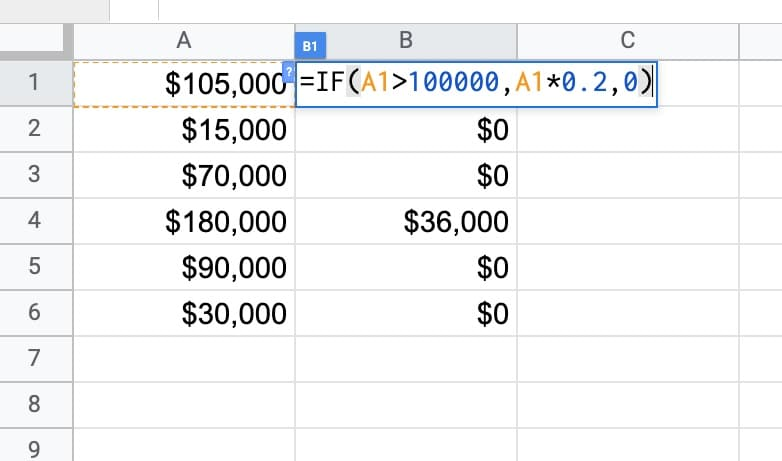## Using The IF Formula for Classification

Another example use case for the IF function is to classify items.

For example, you might want to segment your customers into long-term customers and new customers, based on whether they’ve been a client for 12 months or longer.

Suppose column A was a column containing the number of months a client has been with you, the following IF formula would classify them into long-term or new clients:

`=IF( A1 >= 12 , "Long-term client" , "New client" )`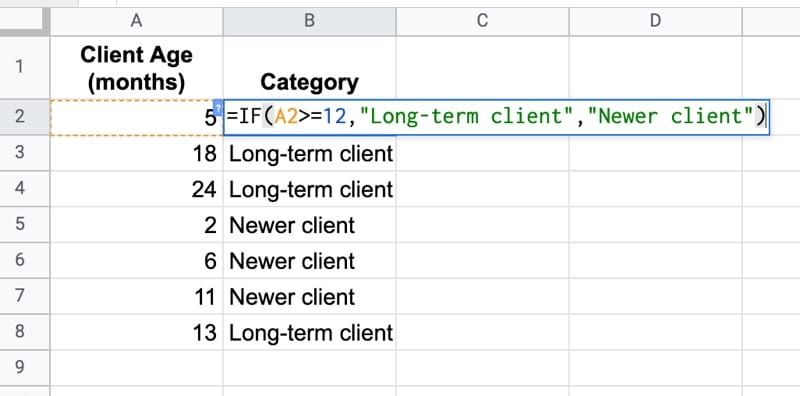This kind of segmentation is useful in lots of different ways.

It lets you compare the retention and churn metrics of the two groups. You can run different marketing campaigns to different segments of your data. Or, maybe you just want to send a “Thank you, you’re awesome!” card to your long-term clients.

## Nested IF Formula in Google Sheets

Sometimes one IF function alone isn’t enough.

Inside the TRUE or the FALSE arguments you can nest another IF function.

It looks complex but if you apply the onion method for working with formulas and work in layers, you’ll see it’s not difficult.

Working from the outside in, it checks if the value is greater than 50. If that is true, then the formula is done and it displays “Greater than 50”.

But if that’s false, it must mean the value is either less than 50 or equal to 50, so we use a second, nested IF to check for that:

`=IF(A2 > 50,"Greater than 50",IF(A2 < 50,"Less than 50","Equal to 50"))`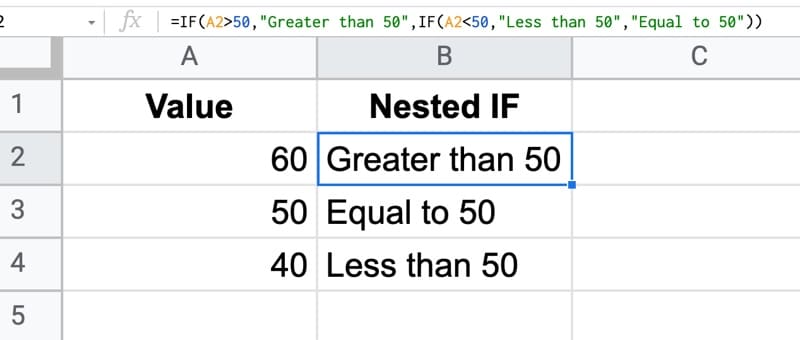## IF Formulas and Checkboxes

Combining checkboxes with IF formulas is a powerful and versatile technique to use in your Sheets.

Checkboxes are actually just TRUE or FALSE values in disguise!

With a checkbox in cell A2, it can only ever have a TRUE or FALSE value. So it can be plugged into the IF function’s first argument directly!

`= IF( A2 , "It's checked!" , "It's not checked." )`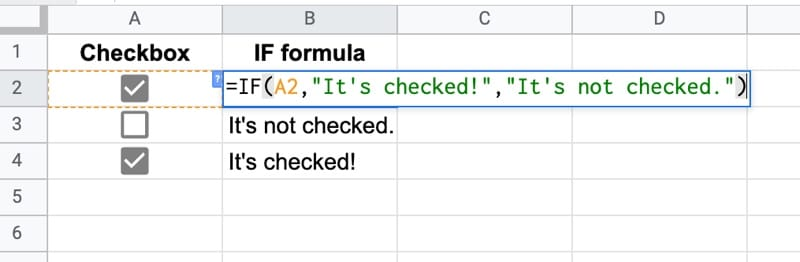This sort of logic is useful in many settings, for example a dashboard where you want to show/hide additional context for specific numbers or charts.

### Direct TRUE or FALSE Input

In the example above, you had a formula already in column A that contained a TRUE or FALSE value.

Since the first argument of the IF statement is looking for a TRUE of FALSE, you only need to reference that cell directly without testing for TRUE / FALSE.

Your IF formula would look like this:

=IF( A1 , “Something true” , “Something false” )

There is NO need to test for TRUE or FALSE again. So you should not see an IF function like this:

=IF( A1 = TRUE , “Something true” , “Something false” )

In fact, you should never see an “= TRUE” or “= FALSE” in an IF statement because they’re redundant.

## Replace numerical IF formulas with MIN or MAX functions

Suppose you have a column of values that you want to cap at a certain level, so that everything above a threshold value, e.g. 200, gets set to that value.

Most of us would approach this by writing an IF formula that checks whether the value is above 200 and then set it to 200 if TRUE, or the actual value if FALSE, like so: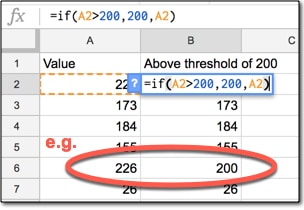However, you can replace the whole IF function with a much more succinct MIN function, which chooses the value 200 if the actual value is larger, since 200 is the minimum:

`=MIN( A2 , 200 )`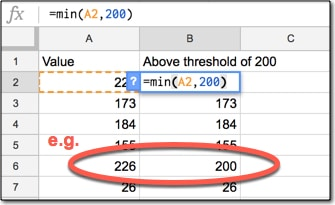Similarly, we can use the MAX function to replace IF statements when we’re looking at a threshold on the low side.

It’s good practice to write efficient formulas because it’s quicker and you’re less likely to make mistakes.

## VLOOKUP Function in Google Sheets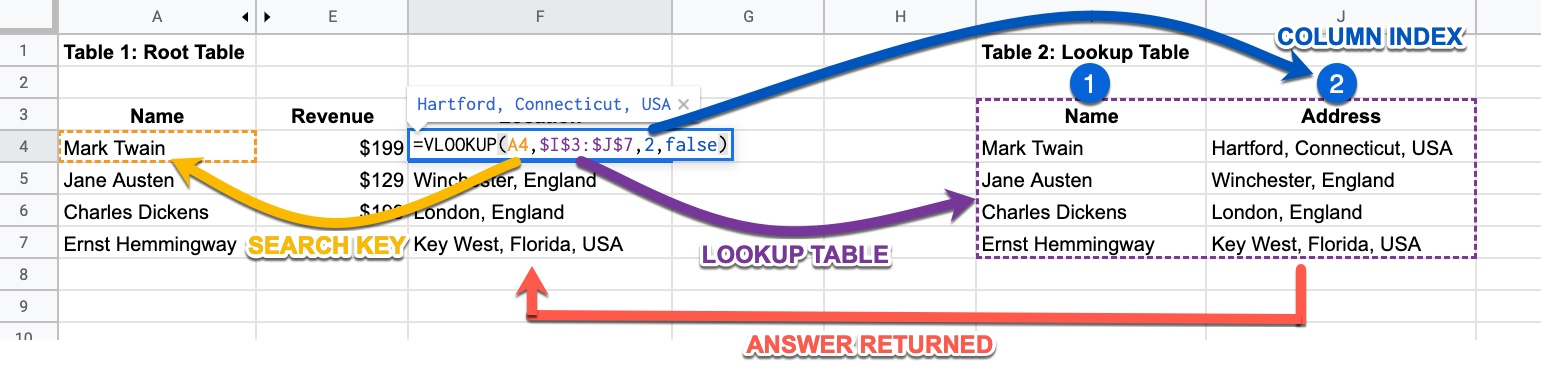VLOOKUP is part of the serious spreadsheet users lingo.

Knowing it signifies you have considerable spreadsheet chops and should be taken seriously.

It’s not difficult to learn if you begin with the concept of the VLOOKUP before getting bogged down in the weeds of the syntax.

And it’s a hundred percent worth your effort to learn. It’s a useful function that you’ll find yourself using frequently once you’ve mastered it.

## What does the VLOOKUP function do?

The VLOOKUP function in Google Sheets is typically used to bring data from one data table and add it into another.

For example, suppose you have a list of customer transactions in one table and a list of customer details in a second table. You might want to add customer data, e.g. location, to the transaction data to do a deeper analysis, e.g. where are my best customers located.

Suppose we run a Saas startup for famous writers, who each pay a monthly fee to subscribe to our service: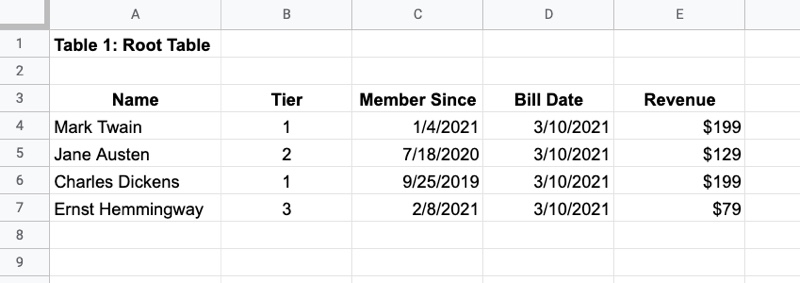In addition, we have a customer table that contains their location details: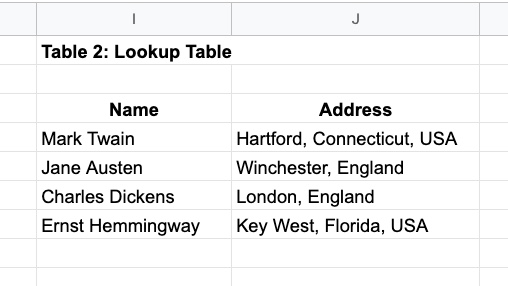We don’t see the full picture if we look at these two tables separately.

If we want to truly understand our sales data, we need to combine these tables so we can see revenue by location.

How would we do this?

We would take each name in turn, which is our Search Term, from the original table. We use the name because it’s in both tables. It’s the common element that lets us link the two tables.

Then we look for that name in the customer table, which is our Lookup Table.

When we find the name we’re looking for, we look across that row to the column containing the information we want to retrieve. In this example we want location, which is column 2. This number is called the Index.

We return the value from column 2 to the relevant row of the original table, which now looks like this: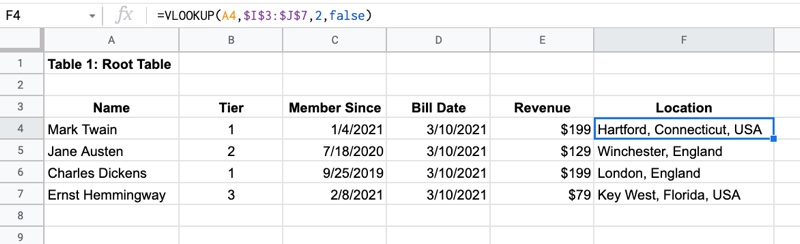Congrats, you’ve just done your first VLOOKUP!

And that’s the VLOOKUP function in Google Sheets in a nutshell.

There’s a lot more nuance than that, but in essence this is what it does.

Although it’s not particularly insightful in this simplified example, it’s not hard to imagine how helpful it is when you have thousands, or tens of thousands, of rows of data.

## VLOOKUP Function in Google Sheets Syntax

Here is the VLOOKUP syntax:

`=VLOOKUP(search_key, range, index, [is_sorted])`

The name VLOOKUP stands for Vertical Lookup. It gets this name because it searches for a value up and down a column, i.e. vertically, rather than horizontally. The horizontal lookup, which is used much less frequently, is called the HLOOKUP.

`search_key`

The search_key is the item from the original table that you want to search for in the lookup table.

`range`

The second argument, range, is the lookup table, i.e. the table we’re going to search in. VLOOKUP searches down the first column of the this table for the search term.

NOTE: VLOOKUP always searches the first column in the lookup table for the search term.

`index`

If and when the search term is found, the value from a specific cell of that row is returned. The index value (the third argument) determines how many columns you go across before returning the value.

For example, index value 1 would just return the search value again (because that’s in the 1st column), index 2 would return whatever is in the adjacent column to the right, index 3 returns the value from the 3rd column of the range, etc.

If the index number is greater than the number of columns in the lookup table (i.e. you’re asking the VLOOKUP formula to return values from column 4 of a lookup table that only has 3 columns), a #REF! error is returned.

`is_sorted`

The final argument, is_sorted, is a TRUE or FALSE value, which you may see written as 1 (TRUE) or 0 (FALSE) occasionally, that indicates whether the column is sorted or not. If it’s omitted, it defaults to a TRUE value.

It determines whether you want to match the search term exactly or find the nearest match.

If you set the fourth argument to FALSE:

• It does exact matching
• It is not case senstive
• If there are multiple matches, the first match is found and the return content is taken from that row (i.e. if Ben Collins appears twice in the lookup table on row 5 and row 10, then information from row 5 is always returned)
• If no lookup value is found, VLOOKUP returns the #N/A error message
• The FALSE setting is used for 99% of VLOOKUP cases

If you set the fourth argument to TRUE:

• It finds the nearest match that is less than or equal to the search key
• This option is rarely used

### Example VLOOKUP Function in Google Sheets

Here’s an example of a VLOOKUP formula:

`=VLOOKUP( A4 , \$I\$3:\$J\$7 , 2 , false )`

The search term is the value in cell A4, “Mark Twain”.

The lookup table is the range \$I\$3:\$J\$7.

Notice the \$ signs around the range references, which make it an absolute reference and lock the range reference in place. This is often required for VLOOKUPs so that when you drag the formula down your column and apply it to new rows, the lookup table stays locked in place. Use the F4 key to quickly add or remove dollar signs.

The index number is 2, meaning the value from the second column of the lookup table will be returned, i.e. the “Address” column.

The final argument is false, meaning this is an exact match.

## VLOOKUP function template

Feel free to make a copy: File > Make a copy…

If you can’t access the template, it might be because of your organization’s Google Workspace settings. If you click the link and open in an Incognito window you’ll be able to see it.

## Approximate matching using A VLOOKUP Formula with TRUE argument

This VLOOKUP lesson is from Day 6 of my free Advanced Formulas 30 Day Challenge course.

Suppose you know the interest rates at your bank: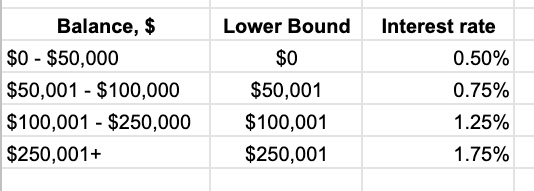And then you have a bunch of accounts for which you want to calculate the interest: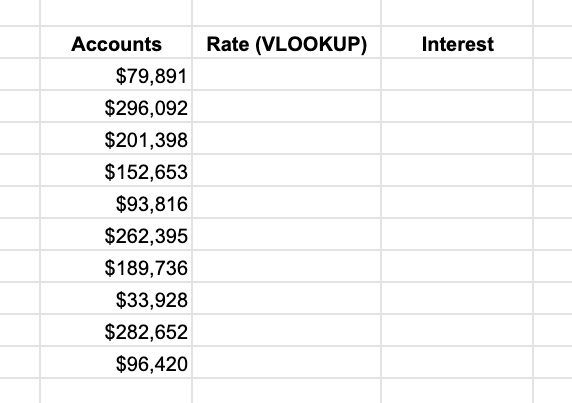It would be tedious to manually lookup each account value in the interest table and determine the rate.

What you can do is use the VLOOKUP with the TRUE argument.

In this example, I have an ordered list of values in column B, from lowest to highest, that denote the different brackets for the interest rate bands.

When I lookup a value (e.g. \$79,891) the VLOOKUP TRUE will search down column B until it reaches a number larger than the search value, then stop on that row before that larger value. In this example, it will stop at \$50,001 because the next value, \$100,001, is larger than the \$79,891. So it returns an interest rate from the \$50,001 row because that represents the \$50,001 – \$100,000 band that the interest rate is in.

It finds the nearest match that is less than or equal to the search key.

The result of the VLOOKUP is: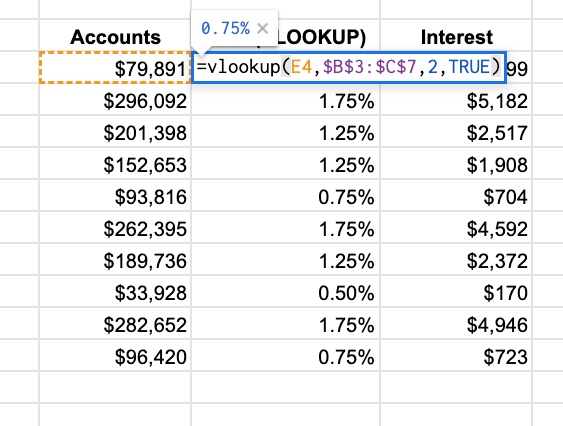## Using The VLOOKUP Function in Google Sheets To Compare Data Lists

Another common use case for VLOOKUP is to compare two lists of data, for example names, and determine what the differences are.

You compare lists by looking for names from list 1 in list 2 and then vice versa, looking for names from list 2 in list 1.

The VLOOKUP formula might look like this:

`=IFERROR( VLOOKUP( A2 , E:E , 1 , false ) , "Not in List 2" )`

• The lookup table is a single column E:E
• So the index must be 1
• With index 1, it returns the name if it’s found
• It uses the IFERROR function to display a custom error message when names are not found

Here is a list comparison example in practice (click to enlarge):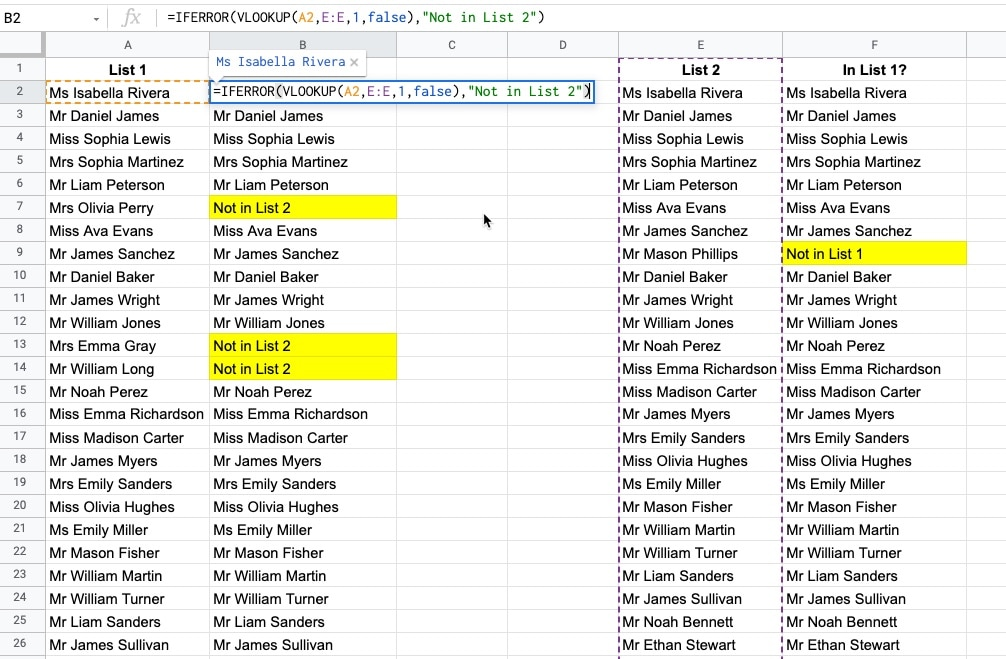VLOOKUP In Google Sheets Using Wildcards For Partial Matches
How to use wildcard characters “*” to perform partial matches in Google Sheets.

How To VLOOKUP To The Left In Google Sheets?
Regular VLOOKUP formulas will only return values from columns to the right of the lookup column. However, there’s a technique using Array Literals that lets us return values from columns to the left of the lookup column.

Have Vlookup Return Multiple Columns in Google Sheets
Have you ever wanted to return more than a single column with VLOOKUP? Perhaps you want to combine two tables and bring all the data across. This tutorial will show you how to do that without requiring multiple VLOOKUP formulas.

VLOOKUP Multiple Criteria in Google Sheets
This tutorial shows you how perform a VLOOKUP using multiple input criteria. For example, you can combine first name and last name inside the VLOOKUP to search against a full name column.